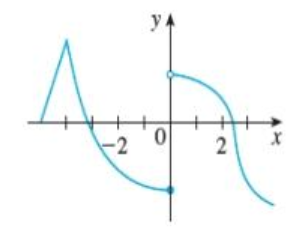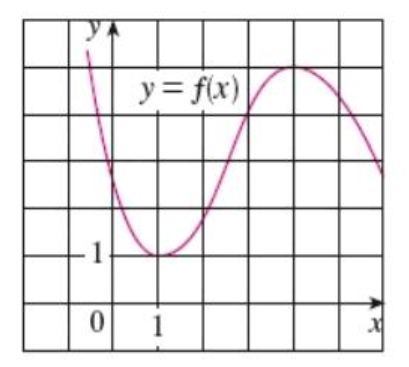# Find: 1. The graph of f is given. State the numbers at which f is not differentiable. Image...

## Question:

1. The graph of {eq}f {/eq} is given. State the numbers at which {eq}f {/eq} is not differentiable.2. Use the given graph to estimate the value of each derivative.

a. {eq}{f}'(0) = {/eq}

b. {eq}{f}'(1) = {/eq}

c. {eq}{f}'(2) = {/eq}

d. {eq}{f}'(3) = {/eq}

e. {eq}{f}'(4) {/eq}## Differentiability And Graph Of Differentiation Of A Given Function:

For any given function f(x), it is differentiable at all the points on its graph except:

1. Where the graph is discontinuous

2. Where it is continuous but the graph has a sharp edge

3. Where it is continuous but has a vertical tangent

The graph of first derivative of a given function {eq}f(x) {/eq} can be constructed using the following information,

1. If {eq}f(x) {/eq} has a maxima or minima at a point then, {eq}f'(x)=0 {/eq} at that point.

2. If {eq}f(x) {/eq} is increasing in an interval then, {eq}f'(x)>0 {/eq} in that interval.

3.If {eq}f(x) {/eq} is decreasing in an interval then, {eq}f'(x)<0 {/eq} in that interval.

1.

The given graph is continuous at all the points except at x=0 where it has a discontinuity. Hence it is not differentiable at this point....

Become a Study.com member to unlock this answer! Create your account